﻿ Flux Theory - flux particle explained

 Properties of Energy & Matter by James Clifford Cranwell 2/17/98 |Abstract|Dimen|Flux|Gravity|Atomic|Relative|Bang|Misc|

Change Font Size: A A A

 by James Clifford Cranwell 2/17/98 Part II ... The Flux

It is well known that all matter in the Universe is composed of atoms and sub-atomic particles, held together by four and only four fundamental forces. These four forces are:

The Strong force (short range) : 100 = 1 = Unit force
This is the force which powers the Sun, is the devastating force behind hydrogen bombs and the force that binds protons / neutrons into nuclei. This unit force is given the value of one, or ten to the power of zero (100), all other forces are measured as a relative strength of it.

Electro-magnetic force (long range): 10-2
This is the force that binds atoms and molecules together and is also the force of electricity. The source of power behind for instance a light bulb. It is one hundredth the strength of Unit force so, the value is 1/100 or one divided by ten to the power of two = 1/(102) = 10-2

Weak force (short range): 10-9
This is the force responsible for radioactive decay and instability in certain nuclei. It is one billionth strength of Unit force so, its value is 1/1,000,000,000 or one divided by ten to the power of nine = 1/(109) = 10-9

Gravitational force (long range): 10-38
This is the force that holds the Earth in orbit around the Sun, keeps our feet firmly anchored to the ground and makes apples fall from trees. It is 1/(1038) (one divided by one followed by 38 zeroes) 1/100,000,000,000,000,000,000,000,000,000,000,000,000 strength of Unit force so, the value is one divided by ten to the power of 38 = 1/(1038) = 10-38

The Gravitational force between any two objects for example the Earth and Moon can be measured using one of Newton's equations F = Gm1m2/r 2 . The constant G in this instance is the Universal gravitational constant. Meaning the force of gravity equals the product of any two masses divided by their radius (separation) squared, quantity times G.
The value of the Gravitational constant is 6.67259 E-11 ...m3 kg-1 s-2
The exact value for this is G = 1/(10 * ?26 -1)c ...m3 kg-1 s-2
G = 1/(10 * ?26 - 1)c = 6.6725903807971 E-11 m3 kg-1 s-2 Exactor
In this instance the speed of light "c" is just an amount of meters or a specific number , not meters per second.
Which gives us the very intriguing...perfectly repeating...continued fractional form ...
1/(10 * ?26 - 1)c= 1 / (10 [5,10,10,10,10,10,10,10,10,10,10,...10 ]-1)c
that fits in really nice in our ten dimensional Universe.
Square root 26 = [5,10,10,10,10,10,10,10,10,10,10,...10 ]
that's 5 and 1/10 with another 1/10 tacked onto the ten in the denominators forever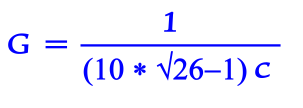```         1
5 + ----------------
1
10 + --------------
10 + 1/(10 + 1/(10 + 1/10... etc.))
```

#### G = 1/((10 * (26^.5))-1)c#### Flunot... center of axis (cut away view)

...So, this is the exact value for the Universal Gravitational Constant. But more importantly this defines the exact shape of the Unit Flux, it's a particle and by simply altering its shape it becomes anything and everything in this Universe. It has 10 hypotenuse or diagonals joined at their centers, terminating on the vertices of the dodecahedron.
This is the same as twenty radii emanating from a common center called a Flunot...

Arrangement of Axis Unit Flux 10-D
It has ten diagonals, nine with the force of light, one minus the charge or plus gravity (the normal state). They are composed of one dimensional (1-D) infinitesimal width string or tube like objects arranged in a ten dimensional Dodecahedron axes pattern terminating on vertices or the set of twenty points...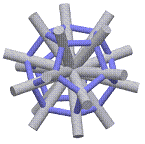```    (+-x/y,  +-xy,     0)  where y = (√5+1)/2
( +-xy,     0, +-x/y)        x = G /(20 * √3)
(    0, +-x/y,  +-xy)        G = 1/(10 * √26 - 1)c
(  +-x,   +-x,   +-x)
```
but, since we are at the quantum level where dimension is viable direction only, that makes ten dimensions in the actual space time continuum. The strings are only one dimension but are arranged in ten dimension Space...so the 10 different axes (20 different directions from origin) occupy or commandeer space in 10 dimensions without actually filling its volumetric area, thereby leaving it almost empty.
The Flux particles set up their own field (face centred cubic spherical space type pack) relative to the object of largest mass in proximity. This corresponds to a lattice which the center of every sphere or particle is the set of all points (x,y,z) where x, y, and z are integers adding up to an even number (That's arrangement, not size).

Flux particle...Nine Dimensional dispersion or 10-9One axis is used to convey the force...either photonic or gravitational, the other Nine axes are one dimension each.
This is a ten dimensional Universe so, every axis is a power of ten. When a force or vibration is being transmitted through one axis of any particle it causes the 18 remaining radii repel away from the charge. As the shape changes from nine Dimension (remaining axes of dodecahedron) weak down to two dimension (disc) electron it causes the particle to separate from the field connections lattice along the way.
The number of field connections is the determinate factor of relative strength or dimension of any particle. Any amount of repel or dimension change is possible here allowing force values from 10-9 through 10-2 on any particle or field created.

Electron...Can disperse energy in 2-dimensions or 10-2 strengthWhen one axis of any particle meets up with a proton charge and is held in place or a large force is being transmitted through it, two of the twenty radii are used as the Unit Force spike. The 18 remaining radii repel away from the charge into a two dimensional disc thus becoming the Electron.
This makes "18" the determinant number in Electron shell arrangements, check the larger noble gases: Argon, Krypton, Xenon, Radon, the amount of electrons in outermost shells will always sum to 18, the first three even have atomic numbers that are multiples of eighteen.
Three groups of six radii from one electron can form (along with seven other electrons) the corners of a cube or the "Octet Rule" (do the math...it works).

Proton...Zero Dimensions or Unit force strength...100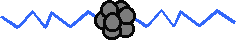With the positive or constricting force the 18 remaining radii curl into six types of Quarks in three flavors thus creating a proton with two string radii left. The flux strings always curl in groups of three (red, blue, green) with combinations of either charge (frequency) or rest (zero) giving the Quark a characteristic One third or Two thirds spin flavor. A group of two is the Proton - Neutron - Connector Meson.
If the proton were the size of the dot above the letter "i" then the strings could be compared to something a lot finer than the web of a spider extending out a few hundred meters, fine enough where eighteen strings can curl into a space the size of the proton and have a spaghetti ball type configuration with a very loose string (or filament) pack.
It is the way to make the most universe with the least amount of material.
Newton's equation F = Gm1m2/r 2 breaks down and becomes ineffective at the proton - electron size or atomic realm, the particle shape has altered and the actual mechanism of attraction or attachment has changed.

Neutron...Nineteen curled radii, one spike left
(dying to curl up with a proton spike)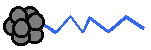The Neutron has one more string radius curl than the proton giving it slightly more mass. Protons can chain together as they curl, a Neutron is a nice way to dead-end the chain so the force of Gravity can ensue.
The string radii or spikes are the mechanism any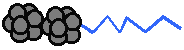particle...electron, proton or neutron etc. uses to connect and relay charge or vibration. In the atomic nucleus the last spike (one radius) of a neutron and one of the two proton spikes curl into a meson, that leaves the whole proton-neutron package with only one spike emanating from the proton, thereby leaving the neutron without an outlet, giving it a neutral charge.
This means an actual free neutron will have all twenty radii curled. If one curled neutron radius suddenly uncoils... it will convert into a proton with the ejecting charge or vibration creating an Electron in the process and is called Beta Radiation (one diameters worth of charge is an Electron or in this case one half... spin?), note: if the curled radius in the proton / neutron connector opposite the proton spike actually breaks off from the proton, once again the neutron radius suddenly uncoils creating the electron and proton but this time there is a free radius or diameter also and is called a neutrino.

Quantum Weirdness:
Electrical energy is used to power mechanical devices but at the quantum level Electrical energy is just a frictionless mechanical vibration.

 Properties of Energy & Matter * part III *